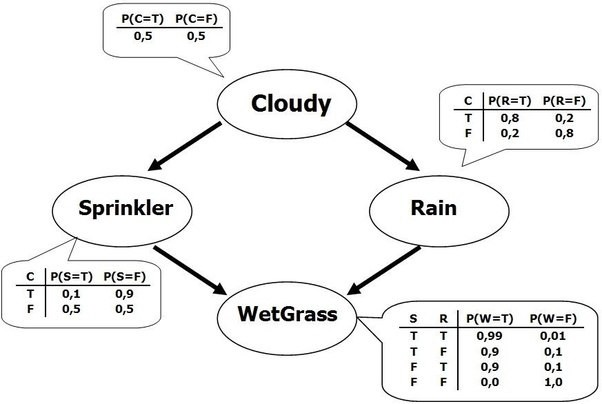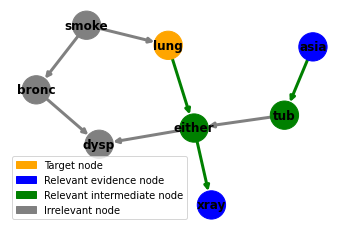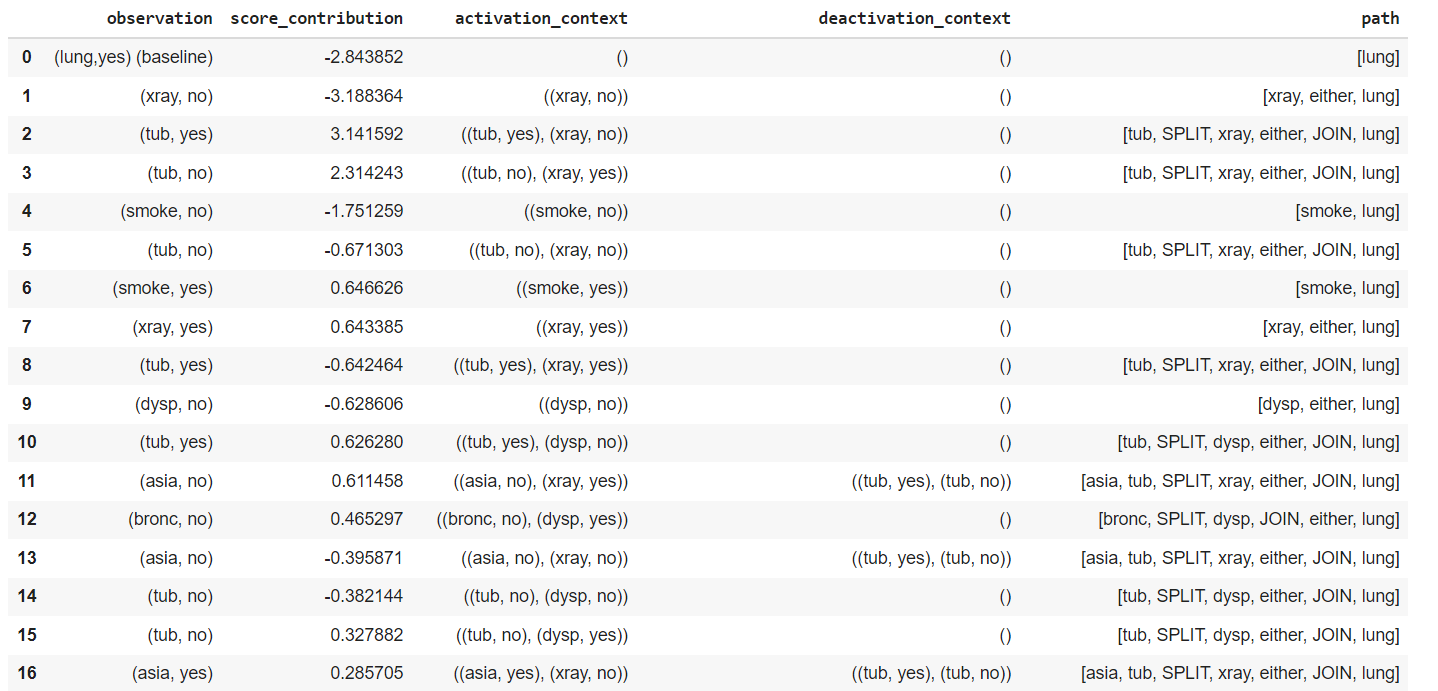# 18

For my PhD research, I have been tinkering with the idea of approximating complex, non-linear statistical models (Bayesian networks) as simple, quasi-linear models.

I am making some headway into the problem, but I feel I need yet to clarify what exactly I want to do, and why this is important.

I am writing this blogpost to 1) spell out the idea better (and get questions and pointers on how to explain it better) and 2) get feedback from the community on which parts of this feel interesting / useful, and which parts I could drop.

First I will explain why I am working on approximating Bayesian networks. Then I will explain the solution I came up with. Finally I will discuss issues with my current approach and next steps.

If you want something to play with, try out this public prototype. It showcases a (clunky) solution to the problem, and might help understand what I am trying to do.

Requisites: you should be familiar with Bayesian Networks, d-separation, belief propagation and vector logodds to understand the gist of this post. Shapley values and double crux are also mentioned in passing, though you should be able to skip the parts where they are mentioned and still follow the post. If there is anything else that seems unclear, you should probably let me know, because it would help me understand what do I need to explain better.

### Theory of research

My (post-hoc) reasoning to have been focusing on this problem is something like this:

• AI models are increasingly embedded in society, and are going to determine an important part of the future of society
• In order to increase the benefits of AI, we need to ensure they are robustly aligned with human values
• One way of going about this is designing ways of making the AI more transparent to users - so they can oversee if the AI is eg not making hiring decisions based on prejudice or making medical diagnosis based on faulty logic
• It is not clear how to do this, but one way of doing it might be 1) identifying how concepts are encoded in AI models, 2) identifying the ways those models process information through the lens of these concepts
• Even if we somehow solve 1), we have that 2) is largely an unsolved problem. Concretely, we have Bayesian models as an example where 1) is solved (by design specially in expertly design networks) but there are no clear concepts and abstractions for 2)
• We do have some transparent, but inefficient ways of doing Bayesian inference (variable elimination). We also have efficient, but opaque ways of doing inference (belief propagation and MCMC). Yet we do not have transparent and efficient ways of doing inference - even though humans seem to do approximate probabilistic inference mostly fine in many circumstances (with some weird glitches)
• More concretely, there are some concrete questions we don’t yet know how to solve regarding the explanation of the output of a Bayesian network. The most important of these are 1) dividing up the ways evidence relates to the output in modular arguments that can be individually considered, 2) quantifying the importance and “direction” of each argument.
• The most relevant past work on this is the system INSITE and its successor BANTER [REF]. They also try to go for this route of identifying “paths of influence”, but their operationalization of argument strength using minimum link strength feels somewhat ad-hoc.
• Other recent work related to this is on Shapley values and friends. However Shapley values work at the “variable” level rather than at the “argument” level, so they don’t help us with for example determining in a network such as in figure 1 if the left path or the right path from top to bottom is more important for the conclusion.Figure 1: a diamond-shape network. Shapley values can tell us the overall importance of the variable Cloudy to determine the value of the variable WetGrass, but it is not entirely obvious how to separate the argument mediated through Sprinkler from the argument mediated through Rain

I think there is a non-trivial chance that I am just fooling myself into thinking that this is something worth pursuing - in my current grant program I have significant pressure to focus on explaining bayesian networks and focus on textual explanations. So here are the reasons why my theory of research might be wrong:

• Whatever I end up learning about Bayesian networks might not transfer to messier architectures.
• Focusing on explaining how information is processed in clean models is a mistake before solving how to identify high level abstractions on messy models, since how we solve the latter problem will largely determine how we solve the former.

I’d be quite interested in hearing other critiques of this theory of research.

### What I have been doing

I have spent the last 6 months thinking about the concrete problem I pointed out above - how to modularize a Bayesian network as a series of arguments and then how to assign importance to each of them. In LessWrong lingo, what I have been doing is a way of extracting cruxes from Bayesian models.

My current approach is something inspired by Rudin et Utsun’s paper on Scoring Systems [REF], combined with belief propagation [REF].

Essentially, what I have been doing is:

1. Find all simple paths from each possible evidence variable in the network to a designated “outcome” variable
2. Use d-separation to list down all conditions where those paths are “open”. This requires the first node of the path to be observed, we need to observe each collider (intermediate node with two incoming arrows) or at least a descendent of it, and to not observe any other intermediate node.
3. Then I do a localized one pass of a modified belief propagation algorithm to study how an observation of the first node in the path would perturb our beliefs about the outcome (this is hard to explain succinctly, see the appendix for details). I quantify this in terms of a logodd change (the “argument score”), and cache the result in a table.
4. I then order all arguments I have studied in decreasing order of absolute logodd change. Intuitively, this corresponds to finding the most important arguments one could make in favor or against an outcome.
5. When presented with some input, I filter the argument table to find all arguments that apply given d-separation rules. I then approximate the final logodds as the baseline logodds of the outcome plus the logodd changes associated with all the arguments that apply.

This is dense and complex, so let’s walk through an example. You can also try out the end result of this process in this interactive notebook.

We will use the Asia network. The outcome we are interested in is whether the patient has lung cancer, which corresponds to the variable lung taking value yes.

1. Finding all possible arguments

We first find all simple (undirected) paths to lung. For example one such path is smokelung. Another more convoluted path is asiatubeitherlung

2. Find the conditions where the arguments apply

Let’s focus on the path asiatubeitherlung. For this path to be open, we need to observe the first node “asia”, and we need to observe the collider either or one of its descendents ie xray or dysp.

This gives rise to 12 instantiations of the argument, eg  (asia=yes, either=yes), (asia=yes, either=no), (asia=yes, xray=yes), (asia=yes, dysp=yes), etc.Figure 3: path asia → tub → either ← lung, where we are using xray → either as context to open the collider either

3. Quantify the importance of each argument

Let’s focus on the argument  asiatubeitherlung when (asia=yes, xray=yes). We run delta belief propagation from asia to tub, then from xray to either, and finally we quantify how the change in tub affects tub given the background change in either due to the change in xray. This sounds convoluted, but the end result is an evidence vector that approximates how much this argument affects lung. We translate this vector into a logodd change, and record it. See the appendix for more info on how this works exactly.

4. Order all the arguments by importance

This is straightforward, given that we already have recorded the importance of each argument in terms of logodd changes. See figure 4 for the result.Figure 4: top identified “arguments” in the ASIA network, ordered by importance (plus baseline on top). So for example, we can see that the xray results are very important for diagnosing lung cancer, since xray-related arguments rank highly in the list. We also see how shorter chains of reasoning are usually stronger, while convoluted ones like asia → tub → either→dysp ← bronc ← smoke → lung are so weak they don’t make it to the top of the list.

5. Apply this for a concrete input

Let’s suppose we observe {'asia': 'no', 'dysp': 'yes', 'bronc': 'no', 'tub': 'no'}

We can then find all arguments that apply under these conditions. So for example the argument tub=noeitherlung  works because we have observed tub=no and dysp=yes to d-open the path. But the argument starting from asia=no does not work, since tub=no blocks the influence of asia on the rest of the diagram.

The former argument has an associated score of 0.3 logodds (as we can see in row 15 of figure 4), so it will shift our credences slightly in favor of the target outcome lung = yes

Because of how delta belief propagation works, we can give explanations of how the evidence propagates along each proposed chain of argument. I have chosen to give just a brief automated textual description, see figure 5 for an example.

### Does this work?

Here there are some results:

• I ran some basic qualitative tests to check that delta belief propagation gives me the results I expected and they do
• The absolute logodd difference between belief propagation and the method I outlined above across all possible evidence inputs is... huhhh… reasonably small (median 0.15 logodds of error) compared to variable elimination in the ASIA network. In other bigger networks like CHILD the results are weirdly off by 0.5 logodds, which suggests a systematic error I do not quite know how to debug
• The visualization kinda looks cool, and the system produces *something*

One problem I have here is that it is not entirely clear what I am aiming to achieve. Some things I could aim for are:

• Accuracy. Getting results that are reasonably close to belief propagation
• Scrutability. Figuring out whether users would be able to identify reasoning mistakes I deliberately introduce in the network.
• Simulability. Whether users can follow and replicate the reasoning of how to apply the scoring table, so that we can hand them the table and have domain experts audit it

### Wrapping up

In summary: I am trying to extract from a Bayesian model the most important considerations on why an input should affect our beliefs about an outcome.

I have a system that does something, but I am confused on how to evaluate it and the grander point of what I am trying to achieve.

Theoretically, I can see some issues with the current framework. The main ones I see are:

1. These scoring tables cannot handle all types of variable interactions, eg they cannot handle noisy OR networks
2. Some arguments are split off in weird ways, eg the argument either=yeslung and that tub=yeseither=yeslung are treated as two different arguments but really it should be treated as one
3. The model is rigid and noninteractive - it doesn’t try to model what things would be surprising to the user. In LessWrong lingo, it exposes cruxes but doesn’t try to double crux.

I have some ideas on how to work on these, but it will be a while until I can make those more concrete and code them up. I’d be interested in other ideas to solve these, and pointers to other issues.

Before I get to address them, I plan to spend some time clarifying what I am trying to do. In order to do that, my first plan is to just demo this system to a bunch of XAI researchers, and see what they have to say about it, plus reading more literature on the goals of the field to see if I can steal any ideas.

### Appendix: delta propagation

The key concept that ties together the algorithm is what I’ve taken to call delta belief propagation. Do not expect a clean explanation because I don’t have one to offer (yet).

Roughly, this is a way of taking an evidence vector  over a variable  and a factor  over variables  and output an evidence vector  over variable , that roughly corresponds to how much of a difference the evidence  makes over , given the probabilistic relations implied by .

Because the input and output of this mecanism is both an evidence vector, it is easy to see how this can be used recursively to study the effect of a path of variables such as the ones we are interested in studying.

How do we actually go about implementing this? My take is to multiply  and , then marginalize all variables but . So far this would be equivalent to belief propagation. However, this mixes the information intrinsically in  together with the information that comes from . To isolate the latter, we divide the result by the result of marginalizing  without multiplying by .

(to address that sometimes we are interested in studying the effect as mediated by a collider, we also allow additional context vectors  that will be multiplied by  before any other computation)

In equation form:

In code form:

 def explain_link(model, source, message, target,
factor, activations={}):

""" explain the effect on target of a change in the beliefs of source
"""

other_nodes = set(factor.variables) - {source, target}

# Contextualize factor
factor = reduce(lambda a,b: a.product(b, inplace=False),
activations.values(),
factor
)
factor.normalize()

f1 = factor.marginalize(other_nodes, inplace=False)
f2 = f1.product(message, inplace=False)

f1.marginalize([source])
f2.marginalize([source])

f1.normalize()
f2.normalize()

delta = f2.divide(f1, inplace=False)
delta.normalize()

assert set(delta.scope()) == {target}, delta.scope()

return delta

Jaime Sevilla is a researcher from Aberdeen University. He is sponsored by the NL4XAI program.

I thank my supervisors Ehud Reiter, Nava Tintarev and Oren Nir for supporting me and encouraging me to write out my ideas.

Mentioned in
New Comment